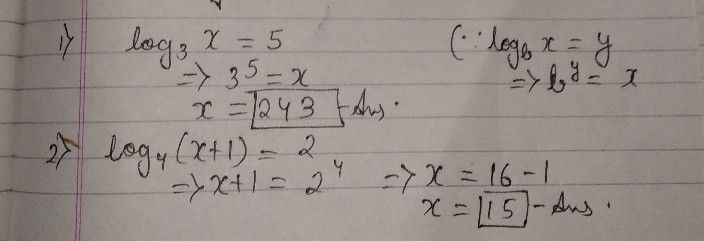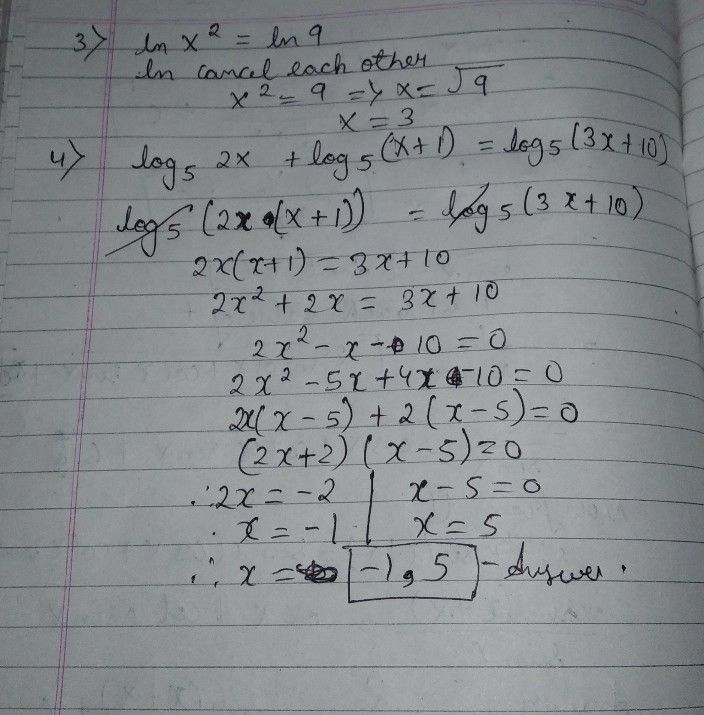Symbol
ProblemExercise Solve the following logarithmic equations. $1.$ $log _{3}x=5$ $2$ $log4\left(x+1\right)=2$ $3$ $lnx^{2}=ln9$ $4$ $log _{5}2x+logs\left(x+1\right)=log _{5}\left(3x+10\right)$
10th-13th grade
Other
Search count: 115
SolutionQanda teacher - Animeshany doubt ask meStudent
thank you teacher